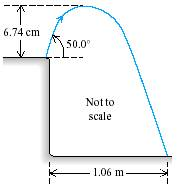# Projectile Motion of a grasshopper off a cliff

#### Todd88

1. The problem statement, all variables and given/known data

A grasshopper leaps into the air from the edge of a vertical cliff, as shown in the figure below.2. Relevant equations

Use information from the figure to find the height of the cliff.

3. The attempt at a solution

The first question was to find the initial speed of the grasshopper. I found this to be 1.5m/s.

I have tried using this equation to get the height of the cliff but it isn't right:

h = ut + (1/2)gt2 where u is the initial velocity. Any help is greatly appreciated.

#### Delphi51

Homework Helper
How did you get the 1.5 m/s?
You must separate the vertical and horizontal motion - they are independent.
Make two headings "horizontal" and "vertical". Write x = vt under the horizontal heading and the accelerated motion formulas under the vertical heading. Fill in all the numbers you have. Note that while you don't know the initial Vx or Vy, you do know their ratio, so enter Vx as an unknown and then Vy as Vx times a tangent. If you have only one unknown in one of the formulas, begin with that! If not, you'll have to use 2 or 3 of them and solve as a system of equations.

Show your equations here if you would like more help!

#### Todd88

Well to get the components of the velocity it is just 1.5sin(50) and 1.5cos(50) so can I just use the y-component and plug that into the formula to find the total distance traveled in the y direction?

#### Delphi51

Homework Helper
Okay, I found the 1.5 too. Tricky calc!
I think you will have to use the horizontal distance first - it matters. Use it to find the time of flight and then use the vertical part to find the vertical distance.

#### Todd88

Okay I got the total time to be 1.0993 s. I used this :

t = 1.06 m / (1.5cos 50)

Not sure where to go to from here...

#### Todd88

Bah, I got it. Just use that time, then the y-component and use the equation:

x = x0 + V0xt + 1/2axt^2

Simple...Thanks a lot for your help!

### The Physics Forums Way

We Value Quality
• Topics based on mainstream science
• Proper English grammar and spelling
We Value Civility
• Positive and compassionate attitudes
• Patience while debating
We Value Productivity
• Disciplined to remain on-topic
• Recognition of own weaknesses
• Solo and co-op problem solving